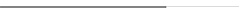## 2021年成人高考专升本高等数学一考试真题及答案

1.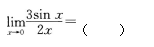A.2/3   B.1   C.3/2    D.3

2.设函数y=2x+sinx,则y/=

A.1-cosx   B.1+cosx   C.2-cosx   D.2+cosx

3.设函数y=ex-2,则dy=

A.ex-3dx   B.ex-2dx    C.ex-1dx    D.exdx

4.设函数y=(2+x）3，则y/=

A.（2+x）2   B.3(2+x)2   C.(2+x)4    D.3(2+x)4

5.设函数y=3x+1,则y/=

A.0   B.1   C.2   D.3

6.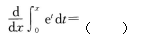A.ex   B.ex-1   C.ex-1    D.ex+1

7.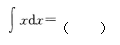A.2x2+C   B.x2+C   C.1/2x2+C   D.x+C

8.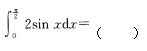A.1/2   B.1   C.2   D.3

9.设函数z=3x2y，则αz/αy=

A.6y   B.6xy    C.3x    D.3X2

10.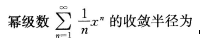A.0   B.1   C.2   D.+∞

11.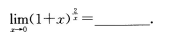12.设函数y=x3,则y/=

13.设函数y=(x-3)4,则dy=

14.设函数y=sin(x-2），则y"=

15.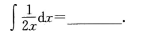16.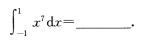17.过坐标原点且与直线（x-1）/3=(y+1)/2+(z-3)/-2垂直的平面方程为

18.设函数x=3x+y2，则dz=

19.微分方程y/=3x2的通解为y=

20.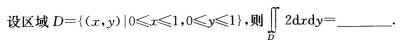21.（本题满分8分）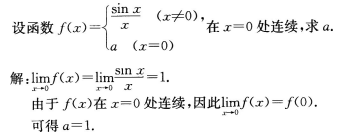22.（本题满分8分）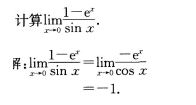23.（本题满分8分）

24.（本题满分8分）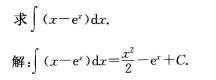25.（本题满分8分）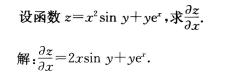26.（本题满分10分）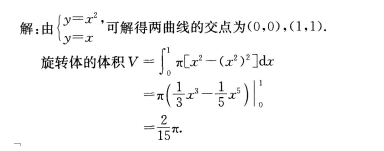## 在线课堂

### 华侨大学夜大学成人高考在线课堂### 华侨大学夜大学自学考试、网络教育在线课堂### 2022年福建省职称（资格）考试在线课堂2022年福建省职称（资格）考试在线课堂 [详细]